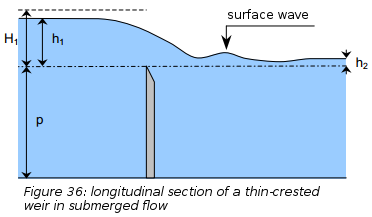# Villemonte 1947

The "Villemonte (1947)2" equation uses the equation of the Free weir to which the flooding coefficient proposed by Villemonte applies (see explanations below). This flooding coefficient is also used for the triangular and truncated triangular weir formulas.Excerpt from CETMEF (2005)1

For a downstream water elevation higher than the crest elevation of the weir, the flow is flooded and a flooding coefficient is applied to the flow coefficient.

Villemonte proposes the following formula:

$K = \frac{Q_{submerged}}{Q_{free}} = \left [ 1- \left ( \frac{h2}{h1} \right)^n \right]^{0.385}$

With:

• $$h_1$$ the upstream water level above the crest of the weir
• $$h_2$$ the downstream water level above the crest of the weir
• $$n$$ the exponent in free flow relationships (rectangular=1.5, triangular=2.5, parabolic=2)

1. CETMEF. Notice sur les déversoirs : synthèse des lois d’écoulement au droit des seuils et déversoirs. Compiègne: Centre d’Études Techniques Maritimes Et Fluviales, 2005. http://www.side.developpement-durable.gouv.fr/EXPLOITATION/DEFAULT/doc/IFD/IFD_REFDOC_0513410/notice-sur-les-deversoirs-synthese-des-lois-d-ecoulement-au-droit-des-seuils-et-deversoirs

2. Villemonte, J.R., 1947. Submerged weir discharge studies. Engineering news record 866, 54–57.